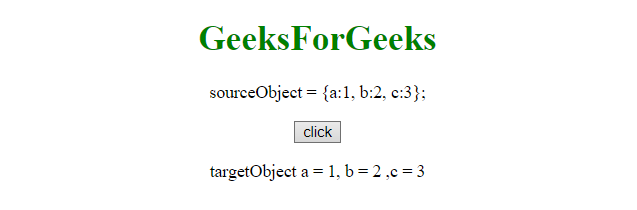# How to clone a JavaScript object?

Cloning a JavaScript object is a task that is used mostly because we do not want to create the same object if the same object already exists.
There are few ways.

• By iterate through each property and copy them to new object.
• Using JSON method as the source object MUST be JSON safe. So it need exception handling to keep it safe in case in which source object can not be convertible to JSON.
• object.assignThis method does only a shallow copy. It means that nested properties are still copied by reference.

Let’s see them one by one with the help of Examples.
Example 1: The one is to iterate through source object’s properties and copy all of them one by one to the target object. It is quite simple but not used very often.

 `   ` `<``html``>   ` `    ``<``head``>  ` `        ``<``title``> ` `    ``  ` `       `  `    ``<``body` `style` `= ``"text-align:center;"``>   ` `        ``<``h1` `style` `= ``"color:green;"` `>   ` `            ``GeeksForGeeks   ` `        ``   ` `        ``<``p` `id``=``"demo2"``>sourceObject = {a:1, b:2, c:3};     ` `        ``<``button` `onClick``=``"fun()"``>click ` `        `` ` `       ``<``p` `id``=``"demo"``>  ` `        ``<``script``> ` `        ``function fun(){ ` `          ``const sourceObject = {a:1, b:2, c:3}; ` `          ``let tO = {}; ` `            ``for (let prop in sourceObject) { ` `              ``if (sourceObject.hasOwnProperty(prop)) { ` `                ``tO[prop] = sourceObject[prop]; ` `              ``} ` `            ``} ` `           ``document.getElementById("demo").innerHTML =  ` `             ``"targetObject a = "+tO.a+", b = " + tO.b+", c = "+tO.c; ` `          ``} ` `        ``  ` `    ``   ` ` `

Output:

• Before we clicked the button:• After clicking the button:• Example 2:This example uses JSON. Using this method, source object MUST be JSON safe.

 `   ` `<``html``>   ` `    ``<``head``>  ` `        ``<``title``> ` `    ``  ` `    ``<``body` `style` `= ``"text-align:center;"``>   ` `        ``<``h1` `style` `= ``"color:green;"` `>   ` `            ``GeeksForGeeks   ` `        ``   ` `        ``<``p` `id``=``"demo2"``>sourceObject = {a:1, b:2, c:3};     ` `        ``<``button` `onClick``=``"fun()"``>click ` `        `` ` `       ``<``p` `id``=``"demo"``> ` `        ``<``script``> ` `        ``function fun(){ ` `          ``const sourceObject = {a:1, b:2, c:3}; ` `          ``let tO = {}; ` `          ``tO = JSON.parse(JSON.stringify(sourceObject)); ` `          ``document.getElementById("demo").innerHTML =  ` `           ``"targetObject a = "+tO.a+", b = " + tO.b+", c = "+tO.c; ` `          ``} ` `        ``  ` `    ``   ` ` `

Output:

• Before we clicked the button:• After clicking the button:• Example 3:This method uses the Object.assign method.

 `   ` `<``html``>   ` `    ``<``head``>  ` `        ``<``title``> ` `    ``  ` `    ``<``body` `style` `= ``"text-align:center;"``>   ` `   `  `        ``<``h1` `style` `= ``"color:green;"` `>   ` `            ``GeeksForGeeks   ` `        ``   ` `        ``<``p` `id``=``"demo2"``>sourceObject = {a:1, b:2, c:3};     ` `        ``<``button` `onClick``=``"fun()"``>click ` `        `` ` `       ``<``p` `id``=``"demo"``>  ` ` `  `        ``<``script``> ` `        ``function fun(){ ` `          ``const sourceObject = {a:1, b:2, c:3}; ` `          ``let tO = {}; ` `          ``tO = Object.assign({}, sourceObject); ` `          ``document.getElementById("demo").innerHTML =  ` `            ``"targetObject a = "+tO.a+", b = " + tO.b+", c = "+tO.c; ` `          ``} ` `        ``  ` `    ``   ` ` `

Output:

• Before we clicked the button:• After clicking the button: